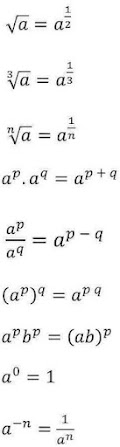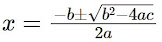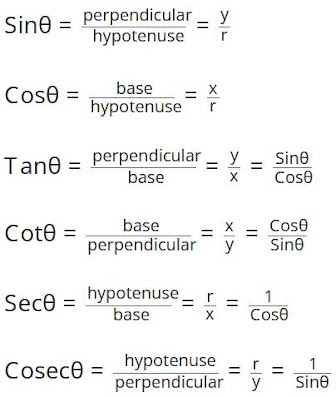# Important Maths Formula for Class 6 to 12 with Solved Questions- Download pdf

Maths formula is very important for the students studying in CBSE medium. It also adds value to the students who are studying in State Boards. There are many benefits of maths formula. It helps the students in:

• Achieve better scores in board exams and entrance examinations.
• The formulas can help students simplify calculations.
• Save time during revision and solve questions easily in the examination.
• Avoid silly mistakes when solving problems involving direct mathematics formulae.
• Students preparing for competitive exams and board exams need to know math formulas.
• It also helps students in calculating and solving exercises easily.
• Develop the mind of the students and helps in the practice.These maths formula makes the brain of students stronger. By practicing these, they would be able to solve a lot of questions very easily.
 Title Maths Formula for Class 6 to 12 Board CBSE and State Boards Related Study materials NCERT Solutions Revision Notes Solved MCQ (Multiple Choice Questions) Important Questions NCERT Textbooks

By studying the given maths formula, one can be able to achieve good marks in the examination. These mathematics formula are prepared by our experts with much efforts. We have also included solved questions for particular formula. In this way, students would be able to apply the mathematics formula in the questions easily.

## Maths Formula |Class 6 to 12

Chapter wise maths formula are provided by studyrankers which makes the solving of problems easier. Maths formula for Class 6 to 12 include various topics with solved examples. These formula are very much needed to solve the questions. Also, we have provided these maths formula in pdf format. Students can download pdf and use it according to their usage. By looking at these formula, students can make guess to apply which formula while solving their exercises. Solved example provided along with formula is cherry on top.

### Some Important Maths Formula

Here are the list of some important formula from Class 6 to 12 which are commonly used.

#### Algebraic Formulas

1. a² – b² = (a-b)(a+b)
2. (a+b)² = a² + 2ab + b²
3. (a-b)² = a² – 2ab + b²
4. a² + b² = (a-b)² +2ab
5. (a+b+c)² = a²+b²+c²+2ab+2ac+2bc
6. (a-b-c)² = a²+b²+c²-2ab-2ac+2bc
7. a³-b³ = (a-b) (a² + ab + b²)
8. a³+b³ = (a+b) (a² – ab + b²)
9. (a+b)³ = a³+ 3a²b + 3ab² + b³
10. (a-b)³ = a³- 3a²b + 3ab² – b³
11. a4 – b4 = (a – b)(a + b)(a2 + b2)
12. a5 – b5 = (a – b)(a4 + a3b + a2b2 + ab3 + b4)
13. “n” is a natural number, an – bn = (a-b) (an-1 + an-2b +….bn-2a + bn-1)
14. “n” is a even number, an + bn = (a+b) (an-1 – an-2b +….+ bn-2a – bn-1)
15. “n” is an odd number an + bn = (a-b) (an-1 – an-2b +…. – bn-2a + bn-1)
16. (am)(an) = am+n (ab)m = amn
17. If n is a natural number, an – bn = (a – b)(an-1 + an-2b+…+ bn-2a + bn-1)
18. If n is even (n = 2k), an + bn = (a + b)(an-1– an-2b +…+ bn-2a – bn-1)
19. If n is odd (n = 2k + 1), an + bn = (a + b)(an-1 – an-2b +an-3b2…- bn-2a + bn-1)
20. (a + b + c + …)2 = a2 + b2 + c2 + … + 2(ab + ac + bc + ….)

#### Exponent Formula

Laws of Exponents: (am)(an) = am+n ; (ab)m = ambm ; (am)n = amn1. For a quadratic equation ax2 + bx + c = 0 where a ≠ 0, the roots will be given by :2. D = b2 − 4ac is called the discriminant
3. For real and distinct roots, D > 0
4. For real and coincident roots, D = 0
5. For non-real roots, D < 0
6. If α and β are the two roots of the equation ax2 + bx + c = 0 then,
α + β = -b/a
α × β = c/a
7. If the roots of a quadratic equation are α and β, the equation will be (x − α)(x − β) = 0

#### Trigonometry Formula

There are total six trigonometrical ratios. They are:

1. sine (sin)
2. cosine (cos)
3. tangent (tan)
4. secant (sec)
5. cosecant (cosec)
6. cotangent (cot)Trigonometry Table

 Angle 00 300 450 600 900 sin θ 0 1/2 1/√2 √3/2 1 cos θ 1 √3/2 1/√2 1/2 0 tan θ 0 1/√3 1 √3 ∞ cot θ ∞ √3 1 1/√3 0 sec θ 1 2/√3 √2 2 ∞ cosec θ ∞ 2 √2 2/√3 1

Trigonometry ratios formula

1. tan θ = sin θ/cos θ
2. cot θ = cos θ/sin θ
3. sin θ = 1/cosec θ
4. cos θ = sin θ/tan θ = 1/sec θ
5. sec θ = tan θ/sin θ = 1/cos θ
6. cosec θ = 1/sin θ
7. sin (90°-θ) = cos θ
8. cos (90°-θ) = sin θ
9. tan (90°-θ) = cot θ
10. cot (90°-θ) = tan θ
11. sec (90°-θ) = cosec θ
12. cosec (90°-θ) = sec θ